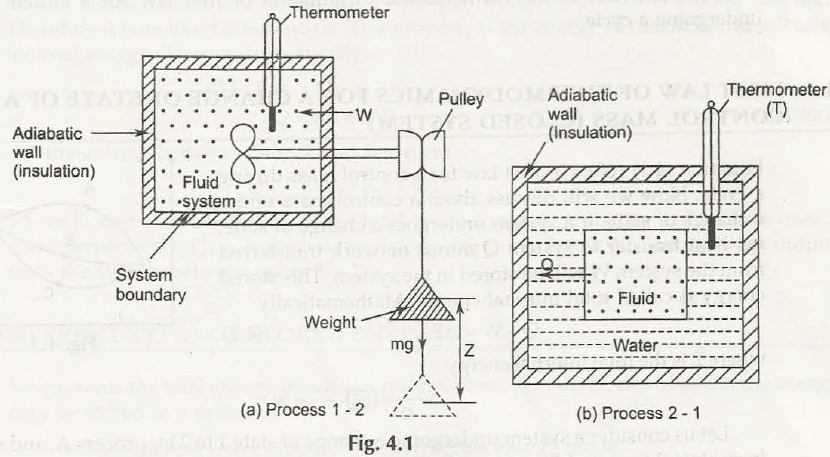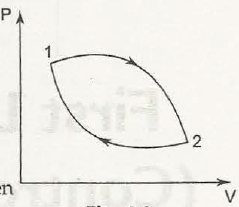A system performs through a cycle that is made of two different processes. In case of first process it would work on system through paddling which will help turn only if the weight is lowered. If the system returns to initial state then transfer heat from one system until cycle gets completed.

The work is measured through mechanical units of force times distance and it is measured through joules and foot pounds force and heat would finally be measured through thermal units that are symbolized as British thermal unit (Btu).

The work and heat measurement is done only during a cycle for different types of system and for various amounts of heat and work. While the amount of heat and work compared, it is visualized that they appears to be proportional. This observation helps us to retrieve the formula related to first law of thermodynamics:

(Eq1)
 J ∮ δQ = ∮ δW

First law of thermodynamics for closed system undergoing a cyclic process

The transfer of heat as well as performance of work in both cases there will be the same effect in system. Energy would get into system as heat way leave the system as work and energy would has entered as work would transfer into heat. The law of conservation of energy would say that the net work performed by system is equal to that of net heat supplied to system.

When any system goes through a thermodynamics cyclic process, then the heat get supplied to system from surrounds which is equal to work done by system on surroundings.Links of Next Mechanical Engineering Topics:-### Customer Reviews

My Homework Help
Rated 5.0 out of 5 based on 510 customer reviews at